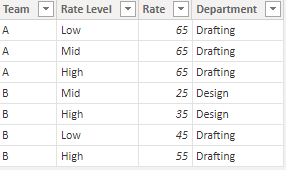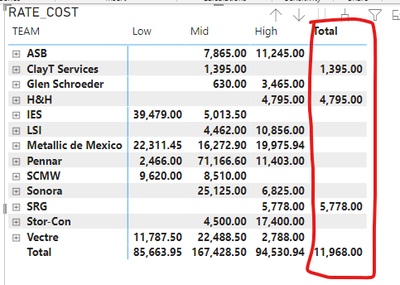cancel
Showing results for
Did you mean:Helper II

## MATRIX, Missing Data on Column Subtotals

Hi there,

I have this matrix that is built from a DAX that sums the total hours (Table A) spend for each team and multiplied it by the cost by hour of that respective team (Table B, see snip) and is split by complexity level and by Department. A single Team can have a different rate either by complexity or by Department.The matrix also has the measure that gives me the right total for each column. Everything fine so far but, as you can see on the snip, some of the total in the Column Subtotals did not appear (highlighted in red), and the cell in green also did not show up although there are hours and rates assigned for that category and the calculation is done it (see second snip).The thing is the cells that are not showing up are the ones that have different rates for each department or Complexity, and I assume that the system chooses not to guess which one shows but:

1- there is a way to force the figure to show up???

2- there is a way to choose/ manipulate with Dax or similar the totals that show on the right of the matrix (green rectangle) in the same way that we can choose what to see on the lower ones (red rectangle) that use a measure like

RATE COST RIGHT TOTAL =
SUMX( VALUES('All Activity  All Nodes'[TEAM]), [RATE COST II] )1 ACCEPTED SOLUTIONSolution Sage

Without seeing the model I am just guessing, but a few things I would be looking at;

Which table are the hours in? Based on what you have provided I am guessing 'All Activity AllNodes' table.

If that is correct, have you created the rate lookup column in the 'All Activity AllNodes' table? And if so, does each row have a value that is not blank?
If each hour line has a coresponding rate value then a sum function should work as long as the fields being added to the visual have appropriate relationships to the 'All Activity AllNodes' table.

If there are blanks in the rate column you will need to troubleshoot why i.e. which combinations of Team, Department and Level are not pulling a rate. Verify the rate exists (which it sounds like you have done already). When looking LOOKUPVALUE in the past I have had small spelling descrepancies mess the whole thing up too.

6 REPLIES 6Community Support

Hi @MSA_BB123 ,

Whether the advice given by @jgeddes  has solved your confusion, if the problem has been solved you can mark the reply for the standard answer to help the other members find it more quickly. If not, please point it out.

Best Regards,
HenrySolution Sage

It is hard to be 100% certain but I believe that you are correct in assuming the issue is the teams with different rates for departments and complexities.
To correct this you have to ensure that each hours row has the correct rate applied to it, then the sums will give you the desired result.

To illustrate I created a small dataset of hours and rates.I then added calculated columns in the hours table that looks up the rate for each row and then multiplies that by the hoursApplied Rate =
LOOKUPVALUE(rateTable[Rate],rateTable[Department],hoursTable[Department],rateTable[Rate Level],hoursTable[Level],rateTable[Team],hoursTable[Team])
Rate Cost =
[Applied Rate] * [Hours]

With each row of hours having the correct rate applied you can now write a sumx measure to use in a visual
Rate Cost Total =
SUMX(hoursTable,hoursTable[Rate Cost])This is a bit of an oversimplified example but hopefully it gets you pointed in the right direction.Helper II

Hi @jgeddes , thanks for reply.

I tried before with a LOOKUPVALUE formula similar that the one you mentioned like this:

Getting the rate II =

LOOKUPVALUE( 'VENDOR-RATES'[RATE],

'VENDOR-RATES'[Team],

SELECTEDVALUE('All Activity  All Nodes'[TEAM]),

'VENDOR-RATES'[RATE LEVEL],

SELECTEDVALUE('All Activity  All Nodes'[LMH Complexity Group]),

'VENDOR-RATES'[Department],

SELECTEDVALUE('All Activity  All Nodes'[DEPARTMENT])

)

and after you mentioned it with a simpler version, since I modified the conection between the tables:

Getting the rate =
LOOKUPVALUE(
'VENDOR-RATES'[RATE]
,'VENDOR-RATES'[CONCAT_COLUMN]
,SELECTEDVALUE('All Activity  All Nodes'[CONCAT_COLUMN])
)

but ironically in my case it shows me even less totals on the COLUMN SUBTOTALSI made sure since the beginning that all the teams have a rate assigned for Design and Drafting otherwise, they would not show up on the result matrix, so that variable has already been considered.

As you mentioned other variables like how the data is set and some others could be modifying my output. Any ideas were to explore???Solution Sage

Without seeing the model I am just guessing, but a few things I would be looking at;

Which table are the hours in? Based on what you have provided I am guessing 'All Activity AllNodes' table.

If that is correct, have you created the rate lookup column in the 'All Activity AllNodes' table? And if so, does each row have a value that is not blank?
If each hour line has a coresponding rate value then a sum function should work as long as the fields being added to the visual have appropriate relationships to the 'All Activity AllNodes' table.

If there are blanks in the rate column you will need to troubleshoot why i.e. which combinations of Team, Department and Level are not pulling a rate. Verify the rate exists (which it sounds like you have done already). When looking LOOKUPVALUE in the past I have had small spelling descrepancies mess the whole thing up too.Helper II

It took me a while but once all the rows with missing RATE assignation where clean the SUM funtion shows the totals.

Thanks!Helper II

Thanks for the suggestion, I'll check that and see if that gives me the expected result.Announcements#### Exclusive opportunity for Women!

Join us for a free, hands-on Microsoft workshop led by women trainers for women where you will learn how to build a Dashboard in a Day!#### Power Platform Conference-Power BI and Fabric Sessions

Join us Oct 1 - 6 in Las Vegas for the Microsoft Power Platform Conference.Top Solution Authors
Top Kudoed Authors
Users online (3,582)